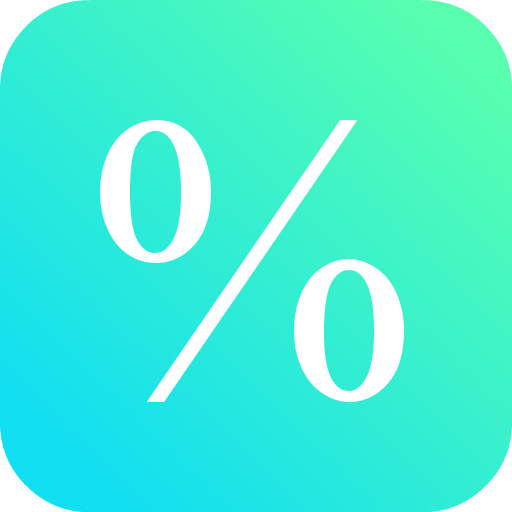# Percent Calculator

نسخه ۳.۹
۵۰
نصب فعال
ابزارها
دسته
۳۹
کیلوبایت
حجم

This free math calculator lets you calculate percentages from a real number.

Best mathematical tool for school and college! If you are a student, it will helps you to learn percent calculations with numbers.

Note: In mathematics, a percentage is a number or ratio expressed as a fraction of 100. It is used to calculate increase and decrease, concentration, interest and so on.